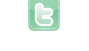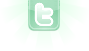## how do you make an object jump

I wonder how got make an object jump can someone help me and send the code in this discussion pls and thx
Will jump always land at the same ground level?
Normal jump basics include something like the following:
```// global
private int ySpeed;

// act
ySpeed++;
boolean onGround = false;
setLocation(getX(), getY()+ySpeed);
Actor actor = getOneIntersectingObject(Actor.class);
if ((actor instanceof Ground) || (actor instanceof Platform))
{
int dir = (int)Math.signum(ySpeed);
setLocation(getX(), actor.getY()-dir*(actor.getImage().getHeight()+getImage().getHeight())/2);
ySpeed = 0;
if (dir > 0) onGround = true;
}
if (onGround && Greenfoot.isKeyDown("up")) ySpeed = -15;```
thanks